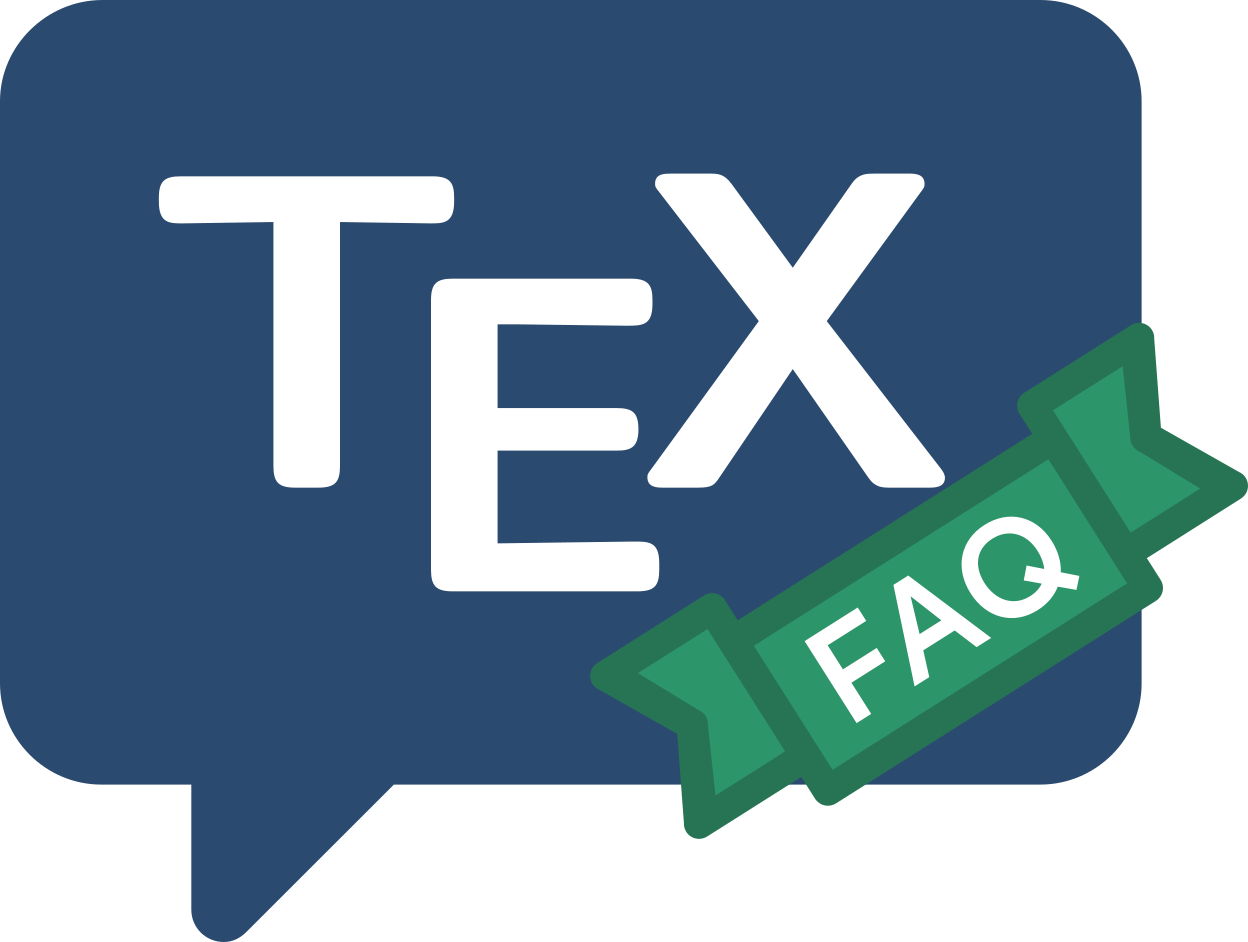Frequently Asked Question List for TeX

# Setting bold Greek letters in LaTeX maths

The issue here is complicated by the fact that \mathbf (the command for setting bold text in TeX maths) affects a select few mathematical “symbols” (the uppercase Greek letters).

In the default configuration, lower-case Greek letters behave differently from upper-case Greek letters (the lower-case greek letters are in the maths fonts, while the upper-case letters are in the original (OT1-encoded) text fonts).

The Plain TeX solution does work, in a limited way; you set a maths style, before you start an equation; thus

{\boldmath$\theta$}


does the job, but \boldmath may not be used in maths mode. As a result, this solution requires that you embed single bold characters in a text box:

$... \mbox{\boldmath$\theta$} ...$


which then causes problems in superscripts, etc. With amsmath loaded,

$... \text{\boldmath$\theta$} ...$


does the trick (and is less bad in regard to superscripts, etc), but is an unsatisfactory solution, too.

These problems may be addressed by using a bold mathematics package.

• The bm package, which is part of the LaTeX tools distribution, defines a command \bm which may be used anywhere in maths mode.
• The amsbsy package (which is part of amsmath bundle) defines a command \boldsymbol, which (though slightly less comprehensive than \bm) covers almost all common cases.

All these solutions apply to all mathematical symbols, not merely Greek letters.

FAQ ID: Q-boldgreek